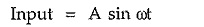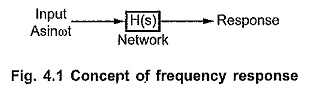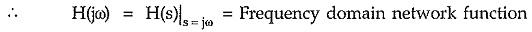## Frequency Domain Analysis:

Frequency Domain Analysis – As we now already, the responses of the networks to the various time dependent inputs such as step, ramp, exponential etc. are studied. Let us now discuss the response by the network to a purely sinusoidal frequency dependent input.

Consider a system with network function H(s). The input applied is purely  sinusoidal and frequency dependent.where

ω = input frequency

The ω is variable and can be changed from 0 to ∞.The response given by the network, when input is purely sinusoidal and its frequency ω is changed over a certain range is called frequency response of the network.

In other words, the steady state response of a network to a variable frequency purely sinusoidal input is called frequency response of a network.

### Frequency Domain Network Function:

To study the effect of change in ω on the network function, it is necessary to obtain frequency domain network function from the s-domain network function.

The network functions which describe the behavior of the networks in the sinusoidal steady state can be obtained by replacing complex frequency variable ‘s’ by ‘jω’. Thus if H(s) is the s domain network function, then frequency domain network function is denoted as H(jω) = H(s)|s=jω.As variable s is complex variable, the frequency domain network function is also a complex function.

Scroll to Top